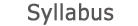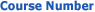0321-2105 Analytical Mechanics2019 - 2020View groups
Course description

Analytical Mechanics – 0321-2105

Lecturer

Prof. Marek Karliner

Email  marek@tauex.tau.ac.il

2019-2020, 1st semeter

Number of Hours/ Credits 4/4

Mandatory/Elective

Mandatory

Prerequisites

0321-1118 – Classical Physics 1

0321-1838 – Mathematical Introduction for Physicists 1

0321-1839 or 0321-1840 – Mathematical Introduction for Physicists 2

Year in program & how often given, if relevant

2nd year, offered annually

Course overview – short abstract

In this course, students become fluent in the use of advanced analytical methods of classical mechanics, mainly in the form of Lagrangian and Hamiltonian mechanics. While doing so, they are introduced to notions such as the analysis of phase-space dynamics, algebraic diagonalization and normal modes of vibrations, canonical transformations, constants of motion, Poisson brackets, action-angle variables, Hamilton-Jacoby theory, and the fundamental connection between symmetry and conservation laws.

Marek Karliner

Learning outcomes – short description (if you don’t have LOs, then don’t write anything in this part)

Described above.

Assignments – 10%

Final exam – 90%

Chapter 1: Introduction to Lagrangian Mechanics

Motivation, generalized coordinates and forces, constraints, virtual work, D’Alembert’s principle, Lagrangian, Hamiltonian, Routhian, Euler-Lagrange equations. Basics of variational calculus and the Euler equation. Action and Hamilton’s principle. Solution to problems with explicit holonomic and nonholonomic constraints. (Chapters 1 and 2 of H&F).

Chapter 2: Analytical Solution of Basic Problems

Stable and unstable mechanical equilibria, vibrations about a stable equilibrium, simple harmonic oscillator with friction and forcing (recap), systems of coupled oscillators, normal modes of vibration and their eigenfrequencies. General analytical solution to one-dimensional problems with a conserved Hamiltonian; simple numerical methods; description of the motion in phase space. Motion in a central potential in three dimensions; conservation of angular momentum; general solution; solution of the Kepler problem; scattering from a central potential, calculation of the differential cross section. (Chapters 3, 4 and 9 of H&F).

Conservation laws and Noether’s theorem. Legendre transformation and Hamilton’s equations. Canonical transformations, generating functions,

Poisson brackets; Hamilton-Jacoby equation; action-angle variables in one dimension. (Chapters 5 and 6 of H&F).

Chapter 4: Rotation and Rigid Body Dynamics

Rotations as orthogonal transformations, vectors, and tensors (recap). Properties of rotating reference frames, fictitious forces (centrifugal, Coriolis, and Euler). Kinetic energy and angular momentum of a rotating rigid body, moment of inertia, Euler equations of motion, free spinning tops, Euler angles, solution of the spinning symmetric top in a gravitational field.  (Chapters 7 and 8 of H&F).

Required text – in language of origin (if Hebrew or Arabic, no need to translate it)

H&F: Analytical Mechanics (Cambridge University Press), L. N. Hand and J. D. Finch.

Recommended reading – in language of origin

Classical Mechanics 2nd or 3rd Editions, (Addison-Wesley), H. Goldstein

Classical Mechanics (University Science Books), J.R. Taylor

Mechanics (Pergamon Press), L.D. Landau and E.M. Lifshitz

Theoretical Mechanics (McGraw-Hill), M.R. Spiegel

Lagrangian Dynamics (McGraw-Hill), D.A. Wells

accessibility declaration